## RD Sharma Class 8 Solutions Chapter 19 Visualising Shapes Ex 19.2

These Solutions are part of RD Sharma Class 8 Solutions. Here we have given RD Sharma Class 8 Solutions Chapter 19 Visualising Shapes Ex 19.2

Other Exercises

Question 1.
Which among the following are nets for a cube ?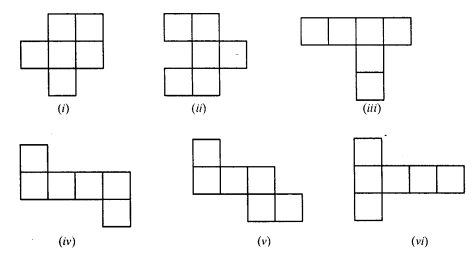Solution:
Nets for a cube are (ii), (iv) and (vi)

Question 2.
Name the polyhedron that can be made by folding each net:Solution:
(i) This net is for a square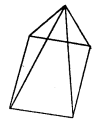(ii) This net is for triangular prism.(iii) This net is for triangular prism.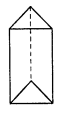(iv) This net is for hexagonal prism.(v) This net is for hexagon pyramid.(vi) This net is for cuboid.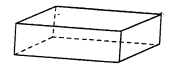Question 3.
Dice are cubes where the numbers on the opposite faces must total 7. Which of the following are dice ?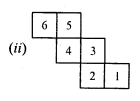Solution:
Figure (i) shows the net of cube or dice.

Question 4.
Draw nets for each of the following polyhedrons: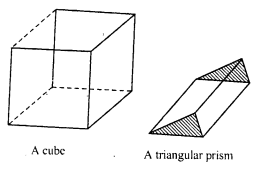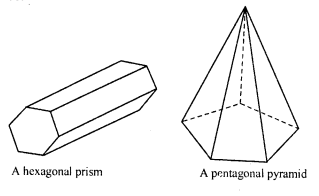Solution:
(i) Net for cube is given below :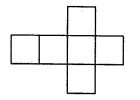(ii) Net of a triangular prism is as under :(iii) Net of hexagonal prism is as under :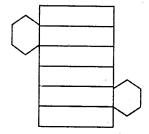(iv) The net for pentagonal pyramid is as under: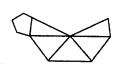Question 5.
Match the following figures: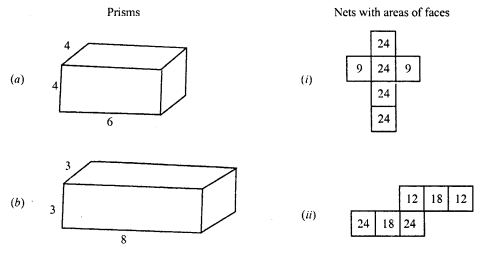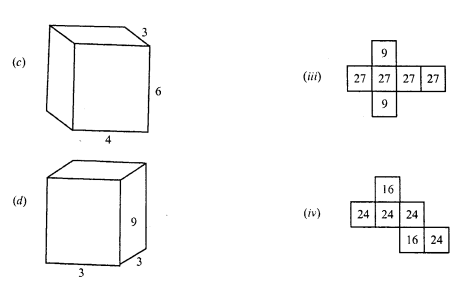Solution:
(a) (iv)
(b) (i)
(c) (ii)
(d) (iii)

Hope given RD Sharma Class 8 Solutions Chapter 19 Visualising Shapes Ex 19.2 are helpful to complete your math homework.

If you have any doubts, please comment below. Learn Insta try to provide online math tutoring for you.